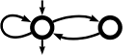Awali Another Weighted Automata library
Quotient

Contains functions related to automata/transducer quotient (in particular, minimisation. More...

## Functions

automaton_t awali::dyn::coquotient (automaton_t aut, std::vector< std::vector< state_t >> &equiv, options_t opts={})

automaton_t awali::dyn::min_coquotient (automaton_t aut, options_t opts={})

automaton_t awali::dyn::quotient (automaton_t aut, std::vector< std::vector< state_t >> &equiv, options_t opts={})
Computes the quotient of an automaton with respect to a given equivalence. More...

## Detailed Description

Contains functions related to automata/transducer quotient (in particular, minimisation.

## ◆ coquotient()

 automaton_t awali::dyn::coquotient ( automaton_t aut, std::vector< std::vector< state_t >> & equiv, options_t opts = `{}` )

Computes the coquotient of an automaton with respect to a given equivalence.

Simply calls transpose , quotient, transpose

Parameters
 aut The automaton whose states should be merged equiv The congruence of mergible states opts A set of options; only SAFE is meaningful. If SAFE is `true`, a test will be performed to verify that `equiv` is indeed a congruence.
Returns
A new automaton with states merged

## ◆ min_coquotient()

 automaton_t awali::dyn::min_coquotient ( automaton_t aut, options_t opts = `{}` )

Computes the minimal coquotient of `aut`. Simply calls transpose, then min_quotient, then transpose.

Parameters
 aut opts A set of options; QUOTIENT_ALGO is meaningful.
Returns
A new automaton.

## ◆ quotient()

 automaton_t awali::dyn::quotient ( automaton_t aut, std::vector< std::vector< state_t >> & equiv, options_t opts = `{}` )

Computes the quotient of an automaton with respect to a given equivalence.

This function merges the states w.r.t. the partition given as second argument.

Argument `equiv` must be a partition: the vectors of states must be disjoint and their union must span over all the states. Only the outgoing transitions of the first state of each part are considered to build the quotient. As a consequence, the result of this function is meaningful only if all equivalent states have the same successors (modulo equiv). A check of this is done unless `opts` sets SAFE to `false`.

Param `equiv` is described as the list of its parts, each part being a list of states; equiv can be partially described: if a state does not appear in any part, it is considered as a singleton part.

Parameters
 aut The automaton whose states should be merged equiv The congruence of mergible states opts A set of options; only SAFE is meaningful. If SAFE is `true`, a test will be performed to verify that `equiv` is indeed a congruence.
Returns
A new automaton with states merged